• No products in the cart.

# 203.5.7 The Neural Network Algorithm

##### Steps of neural network algorithm.

In previous section, we studied about Neural Network Intuition

In all previous posts we progressed to this part. We will breakdown the steps how a neural network starts and ends.

### The Neural Network Algorithm

• Step 1: Initialization of weights: Randomly select some weights .
• Step 2 : Training & Activation: Input the training values and perform the calculations forward.
• Step 3 : Error Calculation: Calculate the error at the outputs. Use the output error to calculate error fractions at each hidden layer
• Step 4: Weight training: Update the weights to reduce the error, recalculate and repeat the process of training & updating the weights for all the examples.
• Step 5: Stopping criteria: Stop the training and weights updating process when the minimum error criteria is met.

Randomly Initialize Weights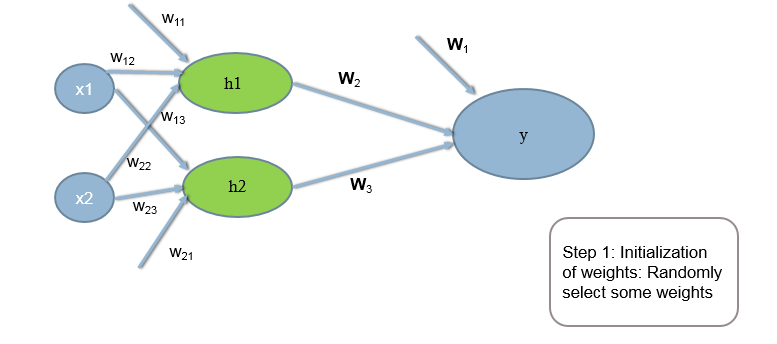Training & Activation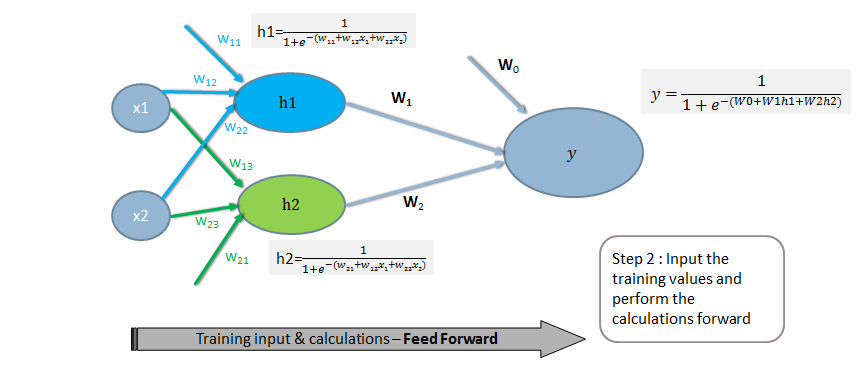Error Calculation at Output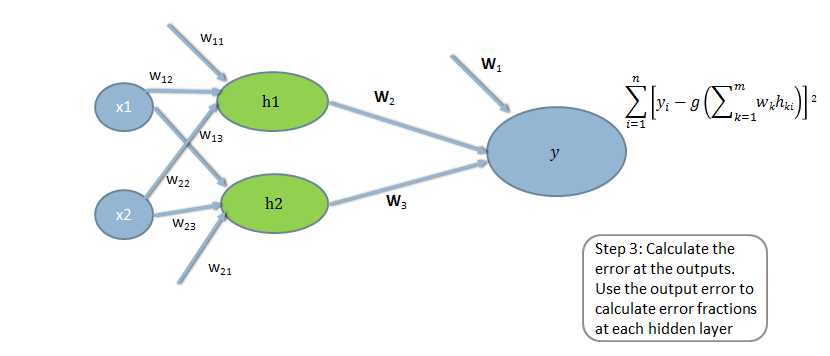Error Calculation at hidden layers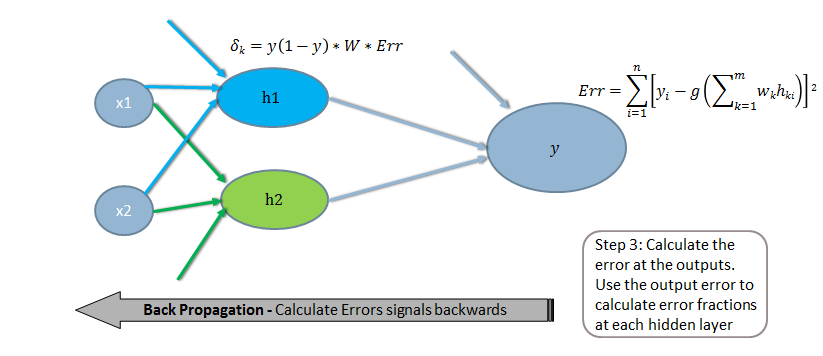Calculate weight corrections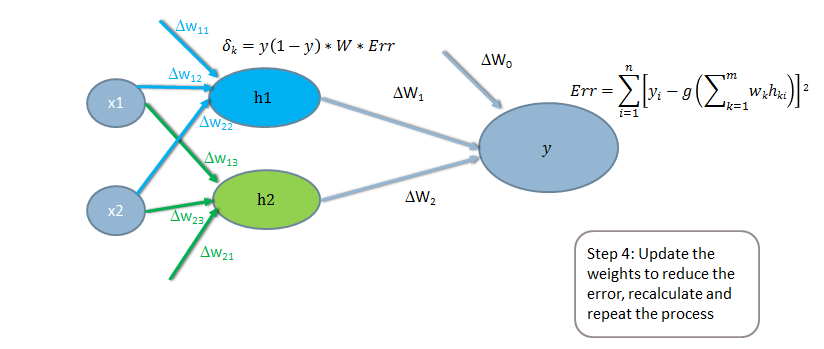Update Weights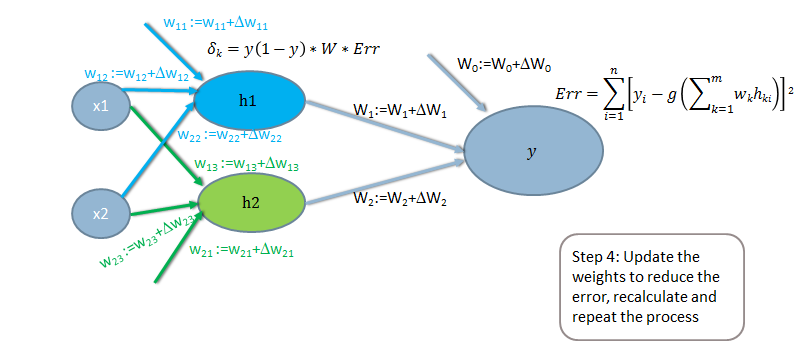Stopping Criteria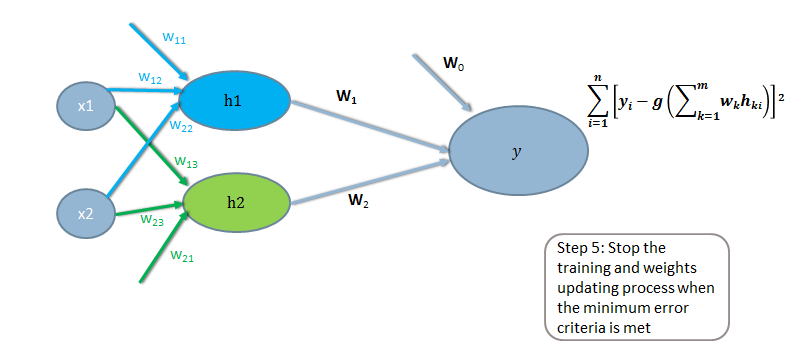The next post is a Demo on Neural Network Algorithm.

21st June 2017